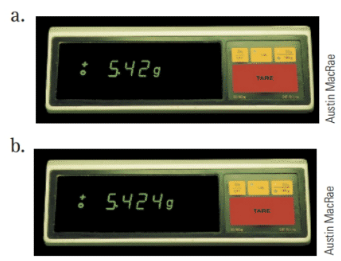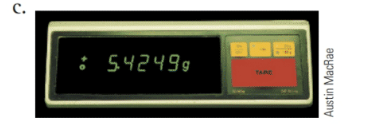# Consider each of the following balances. Which one is the most precise? Which one is the least? How much uncertainty is associated with each balance?### Chemistry In Focus

7th Edition
Tro + 1 other
Publisher: Cengage Learning,
ISBN: 9781337399692

#### Solutions

Chapter
Section### Chemistry In Focus

7th Edition
Tro + 1 other
Publisher: Cengage Learning,
ISBN: 9781337399692
Chapter 2, Problem 52E
Textbook Problem
50 views

## Consider each of the following balances. Which one is the most precise? Which one is the least? How much uncertainty is associated with each balance?Interpretation Introduction

Interpretation:

The most and the least précised balances are to be determined, and hence the uncertainties associated among them are to be examined.

Concept Introduction:

Precision is defined as the closeness of a measurement. More the number of significant figures reported by an instrument, the more precise the instrument is.

Precision of a measurement is calculated by knowing the uncertainty. Uncertainty is the deviation of the measured values from the average value.

### Explanation of Solution

The most precise instrument is (c), the third instrument because it gives the measurement in most number of significant figures. The least precise instrument is (a), the first instrument because it gives the measurement in least number of significant figures.

The average value is calculated as follows:

Average value = (5.42 g + 5.424 g + 5.4249 g3)=5.42 g

### Still sussing out bartleby?

Check out a sample textbook solution.

See a sample solution

#### The Solution to Your Study Problems

Bartleby provides explanations to thousands of textbook problems written by our experts, many with advanced degrees!

Get Started

Find more solutions based on key concepts
Name the following molecular compounds a ClF4 b CS2 c PF3 d SF6

General Chemistry - Standalone book (MindTap Course List)

The most abundant mineral in the body is iron. T F

Nutrition: Concepts and Controversies - Standalone book (MindTap Course List)

2-32 What is the difference between atomic number and mass number?

Introduction to General, Organic and Biochemistry

Show that S1= and S2=i.

Physical Chemistry

In the following diagram, designate each daughter cell as diploid (2n) or haploid (n).

Human Heredity: Principles and Issues (MindTap Course List)

What do the letters in the name MTBE stand for?

Organic And Biological Chemistry

A 75.0-kg firefighter slides down a pole while a constant friction force of 300 N retards her motion. A horizon...

Physics for Scientists and Engineers, Technology Update (No access codes included)

What is a coastal cell? Where does sand in a coastal cell come from? Where does it go?

Oceanography: An Invitation To Marine Science, Loose-leaf Versin